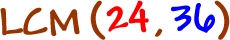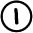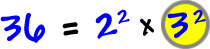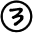Just like GCF's, we can use prime factorizations to find LCM's.  As before, this one is probably at the pre-algebra level.

The Prime Factorization Method:

Let's work with numbers that we've already crunched the prime factorizations for...

 FindList the prime factorization of each using exponents:Take the larger of each of the exponents...Multiply these together to get the answer:Check this using multiple lists!